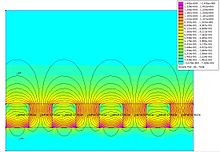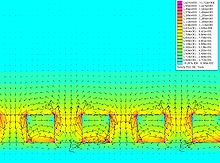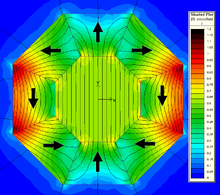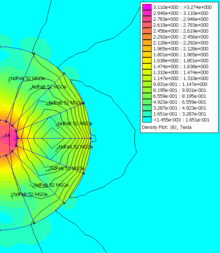# Halbach array

Halbach arrayA Halbach array, showing the orientation of each piece's magnetic field. This array would give a strong field underneath, while the field above would cancel.The flux diagram of a Halbach array

A Halbach array is a special arrangement of permanent magnets that augments the magnetic field on one side of the array while cancelling the field to near zero on the other side. In the diagram, the magnetic field is enhanced on the bottom side and cancelled on the top side (a one-sided flux).

The rotating pattern of permanent magnets (on the front face; on the left, up, right, down) can be continued indefinitely and have the same effect. The effect of this arrangement is roughly similar to many horseshoe magnets placed adjacent to each other, with similar poles touching.

The effect was discovered by Mallinson in 1973, and these "one-sided flux" structures were initially described by him as a "curiosity", although he recognised at the time the potential for significant improvements in magnetic tape technology.

In the 1980s, the late Klaus Halbach, a physicist at Lawrence Berkeley National Laboratory, invented the Halbach array to focus accelerator particle beams.

## Flat Halbach arrays

### Magnetization

Although this magnetic flux distribution seems somewhat counter-intuitive to those familiar with, for example, bar magnets or solenoids, the reason for this flux distribution can be intuitively visualised using Mallinson's original diagram (note this uses the negative y-component, unlike the diagram in Mallinson's paper). The diagram shows the field from a strip of ferromagnetic material with alternating magnetization in the y direction (top left) and in the x direction (top right). Note that the field above the plane is in the same direction for both structures, but the field below the plane is in opposite directions. The effect of superimposing both of these structures is shown in the figure at the bottom:The magnetic field around an infinite halbach array of cube magnets. The field doesn't perfectly cancel due to the discrete magnets used.

The crucial point is that the flux will cancel below the plane and reinforce itself above the plane. In fact, any magnetization pattern where the components of magnetization are$\scriptstyle\frac{\pi}{2}$ out of phase with each other will result in a one-sided flux. The mathematical transform which shifts the phase of all components of some function by$\scriptstyle\frac{\pi}{2}$ is called a Hilbert transform; the components of the magnetization vector can therefore be any Hilbert transform pair (the simplest of which is simply$\scriptstyle\sin(x)\cos(y)$, as shown in the diagram above).

The field on the non canceling side of the ideal, continuously varying, infinite array is of the form:

F(x,y) = F0eikxe ky

Where:$F(x,y)$ is the field in the form$F_x + i F_y$$F_0$ is the magnitude of the field at the surface of the array$k$ is the spatial wavenumber, (i.e., the spatial frequency)$\scriptstyle\frac{2 \pi}{\lambda}$

### Applications

The advantages of one sided flux distributions are twofold:

• The field is twice as large on the side on which the flux is confined (in the idealised case).
• No stray field is produced (in the ideal case) on the opposite side. This helps with field confinement, usually a problem in the design of magnetic structures.

Although one-sided flux distributions may seem somewhat abstract, they have a surprising number of applications ranging from the refrigerator magnet through industrial applications such as the brushless AC motor and magnetic coupling, to high-tech applications such as wiggler magnets used in particle accelerators and free electron lasers. This device is also a key component of the Inductrack Maglev train system wherein the Halbach arrays repel loops of wire that form the track after the train has been accelerated to speed, lifting the train.

The simplest example of a one sided flux magnet is a refrigerator magnet. These are usually composed of powdered ferrite in a binder such as plastic or rubber. The extruded magnet is exposed to a rotating field giving the ferrite particles in the magnetic compound a magnetization resulting in a one-sided flux distribution. This distribution increases the holding force of the magnet when placed on a permeable surface, compared to the holding force from, say, a uniform magnetization of the magnetic compound.

Scaling up this design and adding a top sheet gives a wiggler magnet, used in synchrotrons and free electron lasers. Wiggler magnets wiggle or oscillate an electron beam perpendicular to the magnetic field. As the electrons are undergoing acceleration they radiate electromagnetic energy in their flight direction, and as they interact with the light already emitted, photons along its line are emitted in phase, resulting in a "laser-like" monochromatic and coherent beam.The design shown above is usually known as a Halbach wiggler. The magnetization vectors in the magnetized sheets rotate in the opposite senses to each other; above, the top sheet's magnetization vector rotates clockwise and the bottom sheet's magnetization vector rotates counter-clockwise. This design is chosen so that the x-components of the magnetic fields from the sheets cancel and the y-components reinforce so that the field is given by$H_y \approx \cos(kx)$

where k is the wavenumber of the magnetic sheet given by the spacing between magnetic blocks with the same magnetization vector.

## Halbach cylinder

A Halbach cylinder is a magnetized cylinder composed of ferromagnetic material producing (in the idealised case) an intense magnetic field confined entirely within the cylinder with zero field outside. The cylinders can also be magnetized such that the magnetic field is entirely outside the cylinder, with zero field inside. Several magnetization distributions are shown below:The direction of magnetization within the ferromagnetic material, in plane perpendicular to the axis of the cylinder, is given by$M = M_r\left[\sin(k\phi)\hat{\rho}-\cos(k\phi)\hat{\phi}\right]$

where Mr is the ferromagnetic remanence (T/m). A choice of +k gives an internal magnetic field and -k gives an external magnetic field.

Ideally, these structures would be created from an infinite length cylinder of magnetic material with the direction of magnetization continuously varying. The magnetic flux produced by this ideal design would be perfectly uniform and be entirely confined to the bore of the cylinder. Of course, the ideal case of infinite length is not realisable and in practice the finite length of the cylinders produces end effects which introduce non-uniformities in the field within the bore. The difficulty of manufacturing a cylinder with a continuously varying magnetization also usually leads to the design being broken into segments.

### Applications

These cylindrical structures are used in devices such as brushless AC motors, magnetic couplings and high field cylinders. Both brushless motors and coupling devices use multipole field arrangements:

• Brushless motors typically use cylindrical designs in which all the flux is confined to the centre of the bore (such as k = 4 above, a six pole rotor) with the AC coils also contained within the bore. Such self-shielding motors designs are more efficient and produce higher torque than conventional motor designs.
• Magnetic coupling devices transmit torque through magnetically transparent barriers (that is the barrier is non-magnetic or is magnetic but is not affected by an applied magnetic field), for instance between sealed containers or pressurised vessels. The optimal torque couplings consists of a pair of coaxially nested cylinders with opposite +k and -k flux magnetization patterns, as -k magnetization patterns produce fields entirely external to the cylinder. In the lowest energy state, the outer flux of the inner cylinder exactly matches the internal flux of the outer cylinder. Rotating one cylinder relative to the other from this state results in a restoring torque.

### Uniform fieldsUniform field inside Halbach cylinder

For the special case of k = 2, the field inside the bore is uniform, and is given by:$H = M_r\ln\left(\frac{R_o}{R_i}\right)\hat{y}$

where the inner and outer cylinder radii are Ri and Ro, respectively. H is in the y direction. This is the simplest form of the Halbach cylinder, and it can be seen that if the ratio of outer to inner radii is greater than e the flux inside the bore actually exceeds the remanence of the magnetic material used to create the cylinder.

This cylindrical design is only one class of design which produces a uniform field inside a cavity within an array of permanent magnets. Other classes of design include wedge designs, proposed by Abele and Jensen in which wedges of magnetized material are arranged to provide uniform field within cavities inside the design as shown below.The direction of magnetization of the wedges in (A) can be calculated using a set of rules given by Abele, and allows for great freedom in the shape of the cavity. Another class of design is the magnetic mangle (B), proposed by Coey and Cugat, in which uniformly magnetized rods are arranged such that their magnetization matches that of a Halbach cylinder, as shown for a six rod design. This design greatly increases access to the region of uniform field, at the expense of the volume of uniform field being smaller than in the cylindrical designs (although this area can be made larger by increasing the number of component rods). Rotating the rods relative to each other results in many possibilities including a dynamically variable field and various dipolar configurations. It can be seen that the designs shown in A and B are closely related to the k = 2 Halbach cylinder. Other very simple designs for a uniform field include separated magnets with soft iron return paths, as shown in figure (C).

In the last years, these Halbach dipoles have been used to conduct low-field NMR experiments. Compared to commercially available (Bruker Minispec) standard plate geometries (C) of permanent magnets, they, as explained above, offer a huge bore diameter, while still having a reasonably homogeneous field.

### Varying the field

Halbach cylinders give a static field. However cylinders can be nested, and by rotating one cylinder relative to the other, cancellation of the field and adjustment of the direction can be achieved. As the outside field of a cylinder is quite low, the relative rotation does not require big forces.

## Halbach spheresThe magnetic field in the centre of a halbach sphere can be particularly intense

If the two dimensional magnetic distribution patterns of the Halbach cylinder are extended to three dimensions, the result is the Halbach sphere. These designs have an extremely uniform field within the interior of the design, as they are not affected by the 'end effects' prevalent in the finite length cylinder design. The magnitude of the uniform field for a sphere also increases to 4/3 the amount for the ideal cylindrical design with the same inner and outer radii. However, being spherical, access to the region of uniform field is usually restricted to a narrow hole at the top and bottom of the design.

The equation for the field in a Halbach sphere is:$B = \frac{4}{3} B_0 ln\left(\frac {R_o} {R_i}\right)$

Higher fields are possible by optimising the spherical design to take account of the fact that it is composed of point dipoles (and not line dipoles). This results in the stretching of the sphere to an elliptical shape and having a non-uniform distribution of magnetization over the component parts of the sphere. Using this method, as well as soft pole pieces within the design, 4.5 T in a working volume of 20 mm3 was achieved by Bloch et al. in 1998 and this was increased further to 5 T in 2000[citation needed], although over a smaller working volume of 0.05 mm3. As hard materials are temperature dependent, refrigeration of the entire magnet array can increase the field within the working area further as shown by Kumada et al. This group also reported development of a 5.16 T Halbach dipole cylinder in 2003.

Wikimedia Foundation. 2010.

### Look at other dictionaries:

• Halbach-Array — lineares Halbach Array bestehend aus fünf Segmenten (mit jeweils rotierender magnetischer Orientierung). Ein Halbach Array ist eine spezielle Konfiguration von Permanentmagneten. Eine solche Konfiguration ermöglicht, dass sich der magnetische… …   Deutsch Wikipedia

• Halbach — bezeichnet: einen Familiennamen, siehe Halbach (Familienname) ein Adelsgeschlecht, siehe Bohlen und Halbach (Adelsgeschlecht) einen Ortsteil der Stadt Remscheid, siehe Halbach (Remscheid) Siehe auch: Halbachhammer, verschiedene von Mitgliedern… …   Deutsch Wikipedia

• Matriz Halbach — Una Matriz Halbach o Halbach Array es una combinación especial de imanes permanentes dispuestos para reforzar el campo magnético a un lado de la matriz mientras que elimina por interferencia el campo magnético en el lado opuesto. En el dibujo, el …   Wikipedia Español

• Klaus Halbach — (* 1924 im heutigen Wuppertal; † 11. Mai 2000) war ein deutscher Physiker, der sich mit der Physik von Teilchenbeschleunigern beschäftigte. Halbach wurde an der Universität Basel promoviert und lehrte dann drei Jahre an der Universität Fribourg.… …   Deutsch Wikipedia

• Alfried Krupp von Bohlen und Halbach — Alfried Felix Alwyn Krupp von Bohlen und Halbachcite web url=http://encyclopedia.jrank.org/Cambridge/entries/076/Alfried Alwin Felix Krupp von .html title=Alfried (Felix Alwyn) Krupp (von Bohlen Und Halbach) Biography (1906–67) accessdate=2007 11 …   Wikipedia

• Magnetschienenbahn — Magnetschwebebahn Der später verunglückte Transrapid 08 auf seiner Versuchsstrecke im Emsland Prototyp TR06 vor dem Deuts …   Deutsch Wikipedia

• Inductrack — is a completely passive, fail safe magnetic levitation system, using only unpowered loops of wire in the track and permanent magnets (arranged into Halbach arrays) on the vehicle to achieve magnetic levitation. The track can be in one of two… …   Wikipedia

• Magnetschwebebahn — JR Maglev Magnetschwebebahn – Der später verunglückte Transrapid 08 auf seiner Versuchsstreck …   Deutsch Wikipedia

• Inductrack — ist ein experimentelles Magnetschwebebahn System. Zur Erzeugung des Schwebeeffektes werden Drahtschleifen ohne zusätzliche Stromversorgung in der Gleisanlage und im Fahrzeug in Halbach Arrays angeordnete Raum Temperatur Permanentmagnete verwendet …   Deutsch Wikipedia

• Maglev — JR Maglev at Yamanashi, Japan test track in November 2005 …   Wikipedia